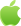# Python如何计算数的阶乘？Python中求阶乘的方法

Python中如何求阶乘？Python中要实现求阶乘的方法有很多，这篇给大家介绍四个方法，分别是使用for循环、使用reduce()函数、使用factorial()函数和使用递归调用。文中的示例代码介绍得很详细，有需要的朋友可以参考，接下来就跟随我一起了解看看吧。```a = int(input('请输入一个整数:'))
num = 1
if a < 0:
print('负数没有阶乘！')
elif a == 0:
print('0的阶乘为1！')
else :
for i in range(1,a + 1):
num *= i
print(num)```

```from functools import reduce
num = reduce(lambda x,y:x*y,range(1,7))
print(num)```

factorial()函数存在math模块下，只有在导入math模块之后，才能对这个函数就那些调用，下面看一个简单的实例，代码如下：

```import math
value = math.factorial(6)
print(value)```

```def num(n):
if n == 0:
return 1
else:
return n * num(n - 1)
print(num(6))```

【原创声明】凡注明“来源：优草派”的文章，系本站原创，任何单位或个人未经本站书面授权不得转载、链接、转贴或以其他方式复制发表。否则，本站将依法追究其法律责任。Python兼职赚钱

2022-03-29 更新Android下载